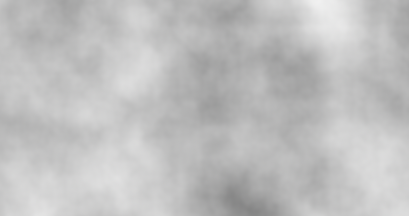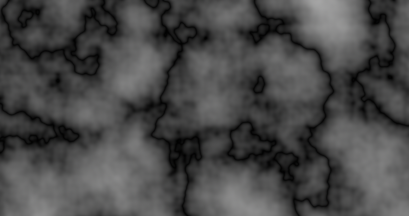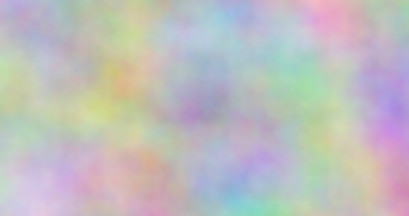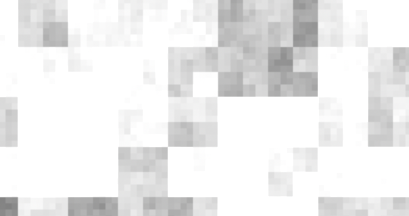# Cloud¶A perlin noise node. This sub-shader computes a classical perlin noise.

## Input

The noise input to use:

• Object will compute the noise using the current 3d position (P) in the object space. The noise will move with the object.
• World will compute the noise using the current 3d position (P) in the world space. The noise will look fix in world space.
• Camera will compute the noise using the current 3d position (P) in the camera space. The noise will be fixed in the camera.
• Screen/Raster/NDC will compute the noise using the current 3d position (P) in the Screen/Raster/NDC spaces, which are projected on the camera.
• st will compute the noise using the first texture coordinate (st). The noise will be fixed in the texture space.
• Offset will compute the noise using only the Offset input.

## Offset

An offset added to the selected noise input. If Input is set to "Offset", this attribute becomes the only noise input.

## Scale

The input value scaling factor.

## Intensity

The scaling factor of the noise result.

## Octaves

Number of noise octaves to use. More octaves add more details.

## Octave Intensity

Control how the Intensity factor is multiplied at each octave. It lowers/increases the high noise frequencies intensity.

## Octave Scaling

Control how the Scale factor is divided at each octave.

## AbsoluteIf checked, computes an absolute noise which render a sharp edge. If unchecked, it computes the classical noise which is smooth.

## RGBIf checked, returns an RGB value, else a greyscale value.

## Cell NoiseIf checked, returns a blocky noise value on every input unit intervals. Else it returns smooth noise values.

## Animate

If checked, animates the noise with the Time value.

## Speed

The animation speed. Not used if Animate is unchecked.